deutsch     english    français     Print

## 8.8 SPECTRAL ANALYSIS### INTRODUCTION

When a beam of light falls on your eyes or you hear a tone, a signal accrues that can be regarded as a function of time y(t). With a single spectral color or a pure tone, it consists of a sinusoidal oscillation with the amplitude A and frequency f, expressed mathematically [more... It does not start counting the time at the beginning of the sine wave, one needs to take into account a phase shift]:

y(t) = Asin(ω * t) where ω = 2 * π * f

 A more complex signal, for example by a constant sustained note of a musical instrument, is still periodic but no longer sinusoidal. The famous mathematician Joseph Fourier (1768-1830) proved that one can also interpret each periodic function as a sum of sine oscillations, as a so-called Fourier series. He thus laid an invaluable foundation for the progress of modern mathematics, physics, and engineering. Breaking a signal down into its sinusoidal frequency components is called spectral analysis..PROGRAMMING CONCEPTS: Sine oscillation, Fourier series, Fast Fourier Transform (FFT), spectrum, sonogram

### SPECTRUM OF A SOUND, OVERTONES

The sinusoidal frequency components that are present are important for the typical tone color of a voice or a musical instrument. A strictly periodic sound consists of the fundamental and the overtones, whose frequencies are integer multiples of the frequency of the fundamental. If you plot the amplitude of the frequency components in a graph, you get the spectrum of the sound. You can determine the spectrum with a device called a spectral analyzer. TigerJython can determine the spectrum using a famous algorithm called the Fast Fourier Transform (FFT).

In order to perform the FFT, you give the function fft(samples, n) a list samples that contains the temporally equidistant sample values and the number n of samples that should be used for the FFT from the beginning of the list.

As return values, you get back a list with the amplitudes of the n/2 (normalized) frequency components. These are separated by the distance r = fs / n, where fs is the sampling frequency. r is (called) the resolution of the spectrum.

These n/2 return values at the distance r "populate" the frequency range from 0 to n/2*r = fs/2, or in a nutshell: The FFT provides the spectrum from 0 to fs/2 at a sampling frequency of fs. A CD with a typical sampling frequency where fs = 44100 Hz corresponds to a spectrum up to 22050 Hz, which covers the entire audible range of humans.

 In order to test the spectrum analyzer, you first use a sound from the distribution of TigerJython named "wav/doublesine.wav" that superimposes two sine tones. The sound was recorded at a sampling frequency of fs = 40,000 Hz. If you take n = 10,000 sampling values, the function fft(samples, n) returns 5'000 frequency components with the resolution r = 40,000 / 10,000 = 4 Hz in the range from 0..20,000 Hz, which you can then display graphically as a spectrum with vertical lines in a GPanel.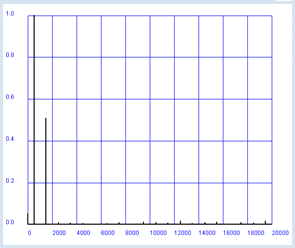```from soundsystem import *
from gpanel import *

def showSpectrum(text):
makeGPanel(-2000, 22000, -0.2, 1.2)
drawGrid(0, 20000, 0, 1.0, 10, 5, "blue")
title(text)
lineWidth(2)
r = fs / n # Resolution
f = 0
for i in range(n // 2):
line(f, 0, f, a[i])
f += r

fs = 40000 # Sampling frequency
n = 10000 # Number of samples
samples = getWavMono("wav/doublesine.wav")
openMonoPlayer(samples, fs)
play()
a = fft(samples, n)
showSpectrum("Audio Spectrum")
```
Highlight program code (Ctrl+C to copy, Ctrl+V to paste)

### MEMO

 As you imagine (and hear) you find the frequencies 500 Hz and 1.5 kHz with an amplitude ratio of 1 : 1/2. There are some additional disturbance components. The frequency 0 corresponds to a constant signal component (offset). You now have a feudal spectrum analyzer in front of you, with which you can examine the fundamentals and overtones of musical instruments, human sounds, or animal sounds with. You will already find the sound of a flue ("wav/flute.wav") and an oboe ("wav/oboe.wav") in the distribution, whose sound characteristics are very different.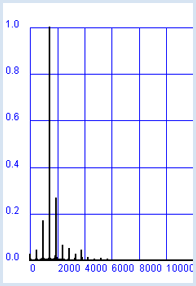### SPECTRA FOR SELF-DEFINED FUNCTIONS

According to the theorem of Fourier, every periodic function with the frequency f can be represented as superpositions of sine functions with the frequencies f, 2*f, 3*f, etc. (Fourier series).

 You can experimentally determine the amplitudes of these frequency components with your Fourier analyzer. Here you consider  a square wave with the frequency f = 1 kHz. The built-in function square(A, f, t) provides you with the value A during the first half of the period and -A in the second. You choose a sampling frequency of fs = 40 kHz and determine the sound samples for a duration of  3s (120'000 values). Then you play the sound clip. For the spectrum, however, you only use 10,000 values and display it.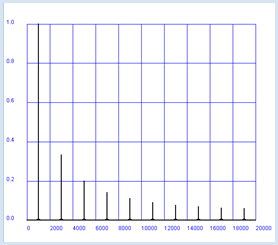```from soundsystem import *
from gpanel import *

def showSpectrum(text):
makeGPanel(-2000, 22000, -0.2, 1.2)
drawGrid(0, 20000, 0, 1.0, 10, 5, "blue")
title(text)
lineWidth(2)
r = fs / n # Resolution
f = 0
for i in range(n // 2):
line(f, 0, f, a[i])
f += r

n = 10000
fs = 40000 # Sampling frequency
f = 1000 # Signal frequency

samples =  * 120000  # sampled data for 3 s
t = 0
dt = 1 / fs # sampling period
for i in range(120000):
samples[i] = square(1000, f, t)
t += dt

openMonoPlayer(samples, 40000)
play()
a = fft(samples, n)
showSpectrum("Spectrum Square Wave")
```
Highlight program code (Ctrl+C to copy, Ctrl+V to paste)

### MEMO

 The experiment shows that the spectrum of a rectangular function consists of the odd multiples of the fundamental frequency and where the amplitudes of the spectral components behave as 1, 1/3, 1/5, 1/7, etc. However, you will never be able to find out experimentally that the spectral parts theoretically stretch until ad infinitum.

### SONOGRAM

FFT is a perfect tool to record the spectral behavior of a sound varying in time, such as a spoken word. Of course in this case, the signal is no longer periodical, but you can assume that it is somewhat periodic piecewise. That is why FFT is often used for short signal blocks, for example for a block length of 100 ms, and repeated every 2.5 ms. Hence, we get a new spectrum for every 2.5 ms that can be represented as a colored vertical line in a sonogram.

 In your program, you start at the beginning of the sampling values and analyze a block length of  2000 values. You begin the next block 50 samples later, etc. In Python, you can do this with a slice operation samples[k * 50:]   where k = 0, 1, 2,... This results in a sonogram, for example for the spoken word "harris", located in the distribution of TigerJython as "wav/harris.wav".```from soundsystem import *
from gpanel import *

def toColor(z):
w = int(450 +  300 * z)
c = X11Color.wavelengthToColor(w)
return c

def drawSonogram():
makeGPanel(0, 190, 0, 1000)
title("Sonogramm of 'Harris'")
lineWidth(4)
# Analyse blocks every 50 samples
for k in range(191):
a = fft(samples[k * 50:], n)
for i in range(n // 2):
setColor(toColor(a[i]))
point(k, i)

fs = 20000 # Sampling freq->spectrum 0..10 kHz
n = 2000 #  Size of block for analyser

samples = getWavMono("wav/harris.wav")
openMonoPlayer(samples, fs)
play()
drawSonogram()
```
Highlight program code (Ctrl+C to copy, Ctrl+V to paste)

### MEMO

 The sonogram shows frequencies in the range from 0..10 kHz vertically, and the course of time from 0 to 190 * 50 / 20000 = 0.475 s horizontally. To convert numbers to colors, you should use the function X11Color.wavelengthToColor() which converts the wavelengths of the color spectrum to colors in the range 380...780 nm. The high spectral components for the sibilant "s" are clearly visible, whereas the fundamentals are entirely missing.

### LIGHT SPECTRA

Light can also be decomposed spectrally in order to determine its contained wavelength components. The wavelengths of the visible spectrum ranges from about 380 nm to 780 nm.It is quite likely that you already know the spectrum analyzer for light, called a prism, which refracts (breaks up) light of various wavelengths at different angles according to the law of refraction.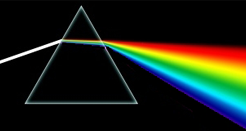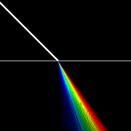In your program, you simulate the transition of a white beam of light in glass and show in a magnification the paths of the different colors.

```from gpanel import *

# K5 glass
B = 1.5220
C = 4590  # nanometer^2
# Cauchy equation for refracting index
def n(wavelength):
return B + C / (wavelength * wavelength)

makeGPanel(-1, 1, -1, 1)
title("Refracting at the K5 glass")
bgColor("black")
setColor("white")
line(-1, 0, 1, 0)

lineWidth(4)
line(-1, 1, 0, 0)
lineWidth(1)

sineAlpha = 0.707

for i in range(51):
wavelength = 380 + 8 * i
setColor(X11Color.wavelengthToColor(wavelength))
sineBeta = sineAlpha / n(wavelength)
x = (sineBeta - 0.45) * 100 - 0.5  # magnification
line(0, 0, x, -1)
```
Highlight program code (Ctrl+C to copy, Ctrl+V to paste)

### MEMO

 If you want to create a beautiful graphic, you should refract the colors more than they would be in real life.

### EXERCISES

 1 Examine other instruments or voices regarding their overtones and try to understand your findings based on the typical character of the sound. 2 Besides the global function square(A, f, t), the functions sine(a, f, t), triangle(A, f, t), sawtooth(A, f, t) are available. Try to guess how the spectrum of a triangular and a sawtooth wave is. You can also analyze the superpositions of sine waves using sine(). 3 In sonograms, analyze the difference between various female and male speakers who say the same word.